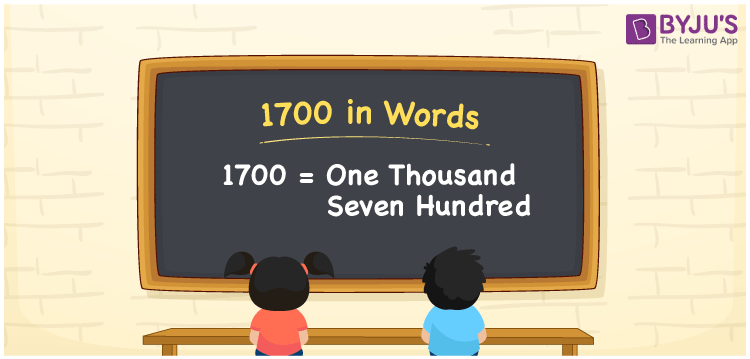# 1700 in Words

1700 in words is written as “One thousand seven hundred”. In Maths, 1700 is a cardinal number that expresses a quantity. If the price of shoes is Rs.1700, then it can be represented as Rupees One thousand seven hundred, in English. Learn more about Numbers In Words at BYJU’S.

 1700 in Words One thousand seven hundred One thousand seven hundred in Numbers 1700

## 1700 in English Words

To make it easy for children to learn the names of the numbers we have represented the chart below. It shows the name of 1700 in English words.## How to Write 1700 in Words?

The number 1700 in words can be written using a place value system, where we can identify the position of each digit in the original number. Since 1700 is a four-digit number, thus, express the digits as:

 Thousands Hundreds Tens Ones 1 7 0 0

From the above table,

1 → Thousands place

7 → Hundreds place

0 → Tens

0 → Ones place

Hence, when we read the number from right to left, it is given by One thousand seven hundred.

### Expanded Form of 1700

We can write the expanded form as:

1 x Thousand + 7 × Hundred + 0 × Ten + 0 × One

= 1 x 1000 + 7 × 100 + 0 × 10 + 0 × 1

= 1000 + 700 + 0 + 0

= One thousand seven hundred

1700 is a whole number that is succeeded by 1699 and preceded by 1701. Learn more about the number 1700 below:

• 1700 in Words – One thousand seven hundred
• Is 1700 an odd number? – No
• Is 1700 an even number? – Yes
• Is 1700 a perfect square number? – No
• Is 1700 a perfect cube number? – No
• Is 1700 a prime number? – No
• Is 1700 a composite number? – Yes

## Frequently Asked Questions on 1700 in words

### What is 1700 in words?

1700 in words is given by One thousand seven hundred.

### What is the rule to write 1700 in words?

1700 in words is written based on the place values of each digit. 1 is at thousand place, 7 at hundred place, 0 at tens place and 0 at ones place. Thus, reading from right to left, we can express 1700 as One thousand seven hundred.

### What is the value of 1700 + 100 in words?

1700 + 100 = 1800, i.e., One thousand eight hundred in words